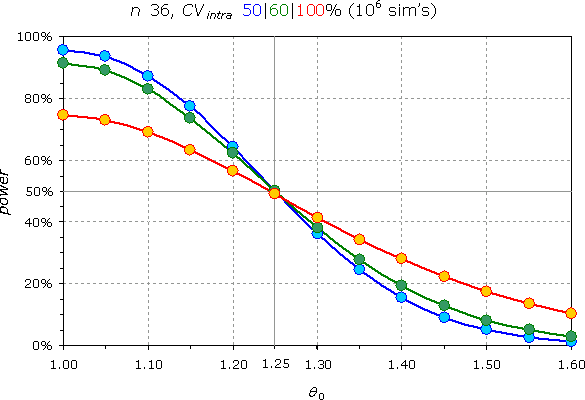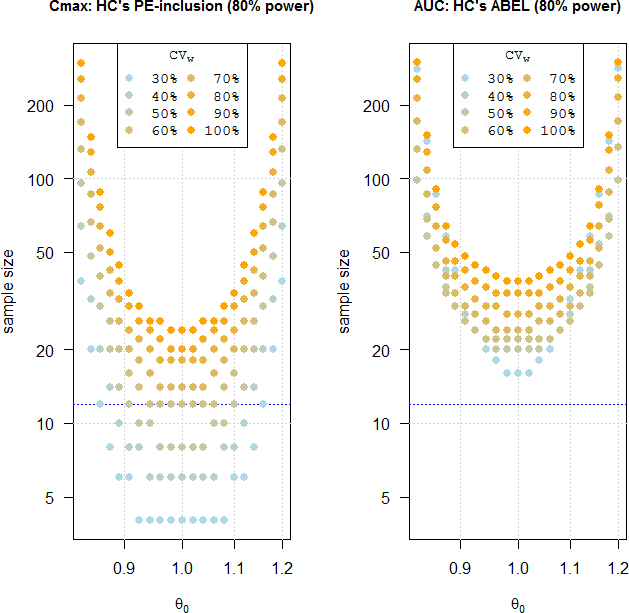Helmut
★★★Vienna, Austria,
2012-12-18 18:04
(3042 d 11:39 ago)

Posting: # 9730
Views: 9,672

## Canada: Sample size for Cmax? [Power / Sample Size]

Dear all,

any idea how to estimate sample size for Cmax (PE within 80–125%; no 90% CI)? Nothing is mentioned in the final guidance; the draft stated in Appendix 1 (lines 752–753):

Sample size calculations, based on a standard where only the mean estimate is required to fall within the bioequivalence interval, are not possible.

Don’t agree. It’s easy enough through simulations (i.e., θ0 0.95, CVintra 40%, n 12, power 82.2%). What puzzles me is the lacking consideration of the patient’s risk. With a θ0 at the limits of the acceptance range by definition 50% of studies will pass – independent from CV and sample size.Reminds me on this goodie.No wonder that HPB didn’t introduce scaling for Cmax.

Dif-tor heh smusma 🖖
Helmut SchützThe quality of responses received is directly proportional to the quality of the question asked. 🚮
Science Quotes
d_labes
★★★

Berlin, Germany,
2012-12-19 14:00
(3041 d 15:43 ago)

@ Helmut
Posting: # 9733
Views: 8,173

## Canada: IUT violated?

Dear Helmut,

» Don’t agree. It’s easy enough through simulations (i.e., θ0 0.95, CVintra 40%, n 12, power 82.2%).

Since no study will decide BE on Cmax alone this is wasting of time I think. The sample size estimation for the other metric AUC will give you with certainty a higher number of needed subjects.

» What puzzles me is the lacking consideration of the patient’s risk. With a θ0 at the limits of the acceptance range by definition 50% of studies will pass – independent from CV and sample size.

As said above no study will decide BE on Cmax alone, but also on AUC.
The decision on AUC has an alpha=0.05. Combining it with the decision on Cmax will not raise the error probability if AUC is truly not BE, I think:
Only a half of the studies which conclude erroneously BE based on AUC will conclude also BE based on Cmax if true ratio for Cmax is on the BE limits. Thus the overall alpha should be <0.05. Correct me if I’m wrong.

But ... In case of 'true BE based on AUC' you are right. Then the overall decision is solely based on the point estimator of Cmax within 80-125%.
Seems the Canadians see this more as a problem of the power of the AUC decision than as a problem of patient’s risk.

Regards,

Detlew
Helmut
★★★Vienna, Austria,
2012-12-29 15:59
(3031 d 13:45 ago)

@ d_labes
Posting: # 9766
Views: 7,797

## Canada: IUT violated?

Dear Detlew,

agree with your points; THX. Excuse my ignorance: IUTDif-tor heh smusma 🖖
Helmut SchützThe quality of responses received is directly proportional to the quality of the question asked. 🚮
Science Quotes
d_labes
★★★

Berlin, Germany,
2012-12-31 13:36
(3029 d 16:07 ago)

@ Helmut
Posting: # 9767
Views: 7,684

## IUT

Dear Helmut,

» IUTNone of them.
I meant: Intersection-Union-Test

That's the crux with acronyms. Everybody uses them, but the meaning is usually restricted to the initiates. But sometimes not even to them.

Regards,

Detlew
VStus
★

Poland,
2017-01-19 10:10
(1549 d 19:34 ago)

@ Helmut
Posting: # 16981
Views: 5,398

## Canada: Sample size for Cmax: R-code?

Dear Helmut,

» Don’t agree. It’s easy enough through simulations (i.e., θ0 0.95, CVintra 40%, n 12, power 82.2%).

Can such a simulation be performed using PowerTOST? If no, can you please give some insight on R-code to do the task?

Thank you very much in advance!
Regards, VStus
zizou
★

Plzeň, Czech Republic,
2017-01-19 12:20
(1549 d 17:24 ago)

@ VStus
Posting: # 16982
Views: 5,410

## Canada: Sample size for Cmax: R-code?

Hi everybody and nobody.

» » Don’t agree. It’s easy enough through simulations (i.e., θ0 0.95, CVintra 40%, n 12, power 82.2%).

Or just trick without simulations with alpha setting to 0.5 (i.e. 1-2*alpha=1-2*0.5=1-1=0).
"0% confidence interval for GMR" is just GMR, I think. x)
Using exact method for power calculation with θ0 0.95, CVintra 40%, n 12, and alpha 0.5 (not 0.05) I can confirm your simulated power 82.2%.

(For θ0=0.80 with alpha=0.5 the power is around 50% as expected.)

» Can such a simulation be performed using PowerTOST? If no, can you please give some insight on R-code to do the task?

Maybe simulations are not required here.

Best regards,
zizou
Helmut
★★★Vienna, Austria,
2017-01-19 12:30
(1549 d 17:14 ago)

@ VStus
Posting: # 16983
Views: 5,607

## HC: Sample size for AUC and Cmax: exact and sim’s

Hi VStus,

» » It’s easy enough through simulations (i.e., θ0 0.95, CVintra 40%, n 12, power 82.2%).
» Can such a simulation be performed using PowerTOST?

Yes – set α to 0.5.library(PowerTOST) alpha <- 0.5 # exact sprintf("%.1f%%", 100*power.TOST(alpha=alpha, CV=0.4, theta0=0.95, n=12)) #  "82.2%" # simulations sprintf("%.1f%%", 100*power.TOST.sim(alpha=alpha, CV=0.4, theta0=0.95, n=12, nsim=1e6)) #  "82.2%"

Or the one of from my OP (1):

library(PowerTOST) CV     <- seq(0.3, 1, 0.1) theta0 <- seq(1, 1.6, 0.005) power  <- matrix(nrow=length(CV), ncol=length(theta0), byrow=TRUE,                  dimnames=list(CV, theta0)) matplot(1, 1, type="n", xlim=range(theta0), ylim=c(0, 100),       xlab=expression(theta), ylab="power (%)",       main="HC\'s PE-inclusion (n=36)", cex.main=1, las=1) grid() ramp   <- colorRamp(c("lightblue", "orange")) col    <- rgb(ramp(seq(0, 1, length=length(CV))), max=255) op     <- par(no.readonly=TRUE) par(family="mono") legend("topright", title=expression(CV[w]),        legend=sprintf("%3.0f%%", 100*CV), lwd=2, col=col, par) par(op) for (j in seq_along(CV)) {  for (k in seq_along(theta0)) {    power[j, k] <- 100*power.TOST(alpha=0.5, CV=CV[j],                                  theta0=theta0[k], n=36)  }  lines(theta0, power[j, ], lwd=2, col=col[j]) }

Coming back to the subject line of this thread (2):

library(PowerTOST) CV     <- seq(0.3, 1, 0.1) hi     <- seq(1, 1.2, 0.02) lo     <- 1/rev(hi) theta0 <- unique(c(lo, hi)) design <- "2x2x4" n.Cmax <- n.AUC <- matrix(nrow=length(CV), ncol=length(theta0), byrow=TRUE,                           dimnames=list(CV, theta0)) for (j in seq_along(CV)) {   for (k in seq_along(theta0)) {     n.Cmax[j, k] <- sampleN.TOST(alpha=0.5, CV=CV[j], theta0=theta0[k],                                  design=design, print=FALSE)[["Sample size"]]     n.AUC[j, k]  <- sampleN.scABEL(alpha=0.05, CV=CV[j], theta0=theta0[k],                                    design=design, regulator="HC",                                    details=FALSE, print=FALSE)[["Sample size"]]   } } ramp   <- colorRamp(c("lightblue", "orange")) col    <- rgb(ramp(seq(0, 1, length=length(CV))), max=255) op     <- par(no.readonly=TRUE) split.screen(c(1, 2)) screen(1) matplot(1, 1, type="n", log="xy", xlim=range(theta0), ylim=range(n.Cmax, n.AUC),      las=1, xlab=expression(theta), ylab="sample size", cex.main=0.9,      main="Cmax: HC\'s PE-inclusion (80% power)") grid() abline(h=12, lty=3, col="blue") par(family="mono") legend("top", title=expression(CV[w]),        legend=sprintf("%3.0f%%", 100*CV), pch=19, cex=0.9,        pt.cex=1.15, col=col, bg="white", ncol=2) par(family="") for (j in seq_along(CV)) {   points(theta0, n.Cmax[j, ], pch=19, cex=1.15, col=col[j]) } screen(2) matplot(1, 1, type="n", log="xy", xlim=range(theta0), ylim=range(n.Cmax, n.AUC),      las=1, xlab=expression(theta), ylab="sample size", cex.main=0.9,      main="AUC: HC\'s ABEL (80% power)") grid() abline(h=12, lty=3, col="blue") par(family="mono") legend("top", title=expression(CV[w]),        legend=sprintf("%3.0f%%", 100*CV), pch=19, cex=0.9,        pt.cex=1.15, col=col, bg="white", ncol=2) par(family="") for (j in seq_along(CV)) {   points(theta0, n.AUC[j, ], pch=19, cex=1.15, col=col[j]) } close.screen(all=TRUE) par(op)Even if the CV of Cmax is much higher than the one of AUC we don’t have to worry about it – confirming what Detlew wrote above.

Now try this one (3):

library(PowerTOST) CV     <- seq(0.3, 1, 0.1) theta0 <- seq(1, 1.2, 0.02) design <- "2x2x4" n.Cmax <- n.AUC <- matrix(nrow=length(CV), ncol=length(theta0), byrow=TRUE,                           dimnames=list(CV, theta0)) ramp   <- colorRamp(c("lightblue", "orange")) col    <- paste0(rgb(ramp(seq(0, 1, length=length(CV))), max=255), "80") matplot(1, 1, type="n", log="xy", xlim=c(6, 300), ylim=c(6, 300),las=1,         xlab="Cmax [PE 80.0\u2013125.0%]",         ylab="AUC [ABEL]", cex.main=1,         main="sample size for 80% power") grid() abline(a=0, b=1, col="gray") op     <- par(no.readonly=TRUE) par(family="mono") legend("bottomright", title=expression(CV[w]),        legend=sprintf("%3.0f%%", 100*CV), pch=19, pt.cex=1.25, col=col,        bg="white", ncol=2) par(op) for (j in seq_along(CV)) {   for (k in seq_along(theta0)) {     n.Cmax[j, k] <- sampleN.TOST(alpha=0.5, CV=CV[j], theta0=theta0[k],                                  design=design, print=FALSE)[["Sample size"]]     n.AUC[j, k]  <- sampleN.scABEL(alpha=0.05, CV=CV[j], theta0=theta0[k],                                    design=design, regulator="HC",                                    details=FALSE, print=FALSE)[["Sample size"]]     points(n.Cmax[j, k], n.AUC[j, k], pch=19, cex=1.4, col=col[j])   } }If the CVs of Cmax and AUC would be equal, ABEL still drives the sample size. At high CVs and PEs off from 1 scaling doesn’t help much more due to the CV cap + PE-restriction. Therefore, even in ABEL mainly the PE-restriction leads the BE-decision.

Edit: Patience! Runtimes on my machine: 1.9 s (1), 33 s (2), 17 s (3).

Dif-tor heh smusma 🖖
Helmut SchützThe quality of responses received is directly proportional to the quality of the question asked. 🚮
Science Quotes
VStus
★

Poland,
2017-01-31 13:44
(1537 d 16:00 ago)

@ Helmut
Posting: # 17001
Views: 4,961

## HC: Sample size for AUC and Cmax: exact and sim’s

Dear zizou,
Dear Helmut,

Thank you very much! At first I though that RStudio got frozen :)

Sorry for not responding earlier, notification has not worked.

In the meantime, Canadian CRO just used Intra-subject variability of AUCt (very low) with extreme T/R to justify the sample size of 24. And we have reasons to assume that real T/R for Cmax is far below unity. This gives less than 60% power on 24 subjects, but still more than just a coin flipAnd almost no one will want to run bioequivalence study on hundreds of subjects...

Regards, VStus
Admin contact
21,419 posts in 4,475 threads, 1,510 registered users;
online 11 (0 registered, 11 guests [including 1 identified bots]).
Forum time: Sunday 06:44 CEST (Europe/Vienna)

Nothing fails like success because you do not learn anything from it.
The only thing we ever learn from is failure.
Success only confirms our superstitions.    Kenneth E. Boulding

The Bioequivalence and Bioavailability Forum is hosted byIng. Helmut Schütz